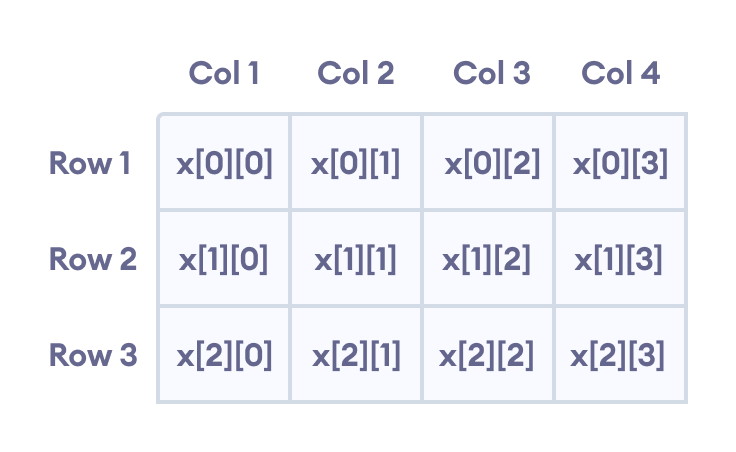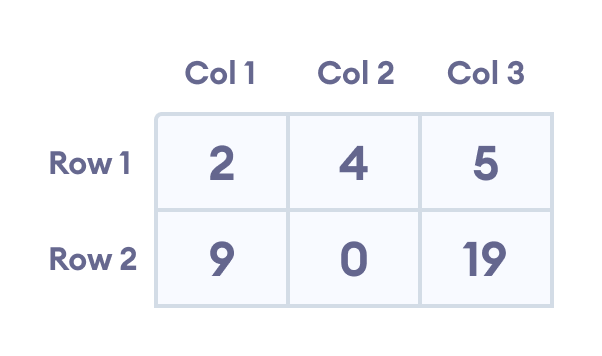# C++ Multidimensional Arrays

In this tutorial, we'll learn about multi-dimensional arrays in C++. More specifically, how to declare them, access them, and use them efficiently in our program.

In C++, we can create an array of an array, known as a multidimensional array. For example:

``int x;``

Here, x is a two-dimensional array. It can hold a maximum of 12 elements.

We can think of this array as a table with 3 rows and each row has 4 columns as shown below.Elements in two-dimensional array in C++ Programming

Three-dimensional arrays also work in a similar way. For example:

``float x;``

This array x can hold a maximum of 24 elements.

We can find out the total number of elements in the array simply by multiplying its dimensions:

``2 x 4 x 3 = 24``

## Multidimensional Array Initialization

Like a normal array, we can initialize a multidimensional array in more than one way.

### 1. Initialization of two-dimensional array

``int test = {2, 4, 5, 9, 0, 19};``

The above method is not preferred. A better way to initialize this array with the same array elements is given below:

``int  test = { {2, 4, 5}, {9, 0, 19}};``

This array has 2 rows and 3 columns, which is why we have two rows of elements with 3 elements each.Initializing a two-dimensional array in C++

### 2. Initialization of three-dimensional array

``````int test = {3, 4, 2, 3, 0, -3, 9, 11, 23, 12, 23,
2, 13, 4, 56, 3, 5, 9, 3, 5, 5, 1, 4, 9};``````

This is not a good way of initializing a three-dimensional array. A better way to initialize this array is:

``````int test = {
{ {3, 4, 2, 3}, {0, -3, 9, 11}, {23, 12, 23, 2} },
{ {13, 4, 56, 3}, {5, 9, 3, 5}, {5, 1, 4, 9} }
};``````

Notice the dimensions of this three-dimensional array.

The first dimension has the value `2`. So, the two elements comprising the first dimension are:

```Element 1 = { {3, 4, 2, 3}, {0, -3, 9, 11}, {23, 12, 23, 2} }
Element 2 = { {13, 4, 56, 3}, {5, 9, 3, 5}, {5, 1, 4, 9} }
```

The second dimension has the value `3`. Notice that each of the elements of the first dimension has three elements each:

```{3, 4, 2, 3}, {0, -3, 9, 11} and {23, 12, 23, 2} for Element 1.
{13, 4, 56, 3}, {5, 9, 3, 5} and {5, 1, 4, 9} for Element 2.```

Finally, there are four `int` numbers inside each of the elements of the second dimension:

```{3, 4, 2, 3}
{0, -3, 9, 11}
... .. ...
... .. ...```

## Example 1: Two Dimensional Array

``````// C++ Program to display all elements
// of an initialised two dimensional array

#include <iostream>
using namespace std;

int main() {
int test = {{2, -5},
{4, 0},
{9, 1}};

// use of nested for loop
// access rows of the array
for (int i = 0; i < 3; ++i) {

// access columns of the array
for (int j = 0; j < 2; ++j) {
cout << "test[" << i << "][" << j << "] = " << test[i][j] << endl;
}
}

return 0;
}``````

Output

```test = 2
test = -5
test = 4
test = 0
test = 9
test = 1```

In the above example, we have initialized a two-dimensional `int` array named test that has 3 "rows" and 2 "columns".

Here, we have used the nested `for` loop to display the array elements.

• the outer loop from `i == 0` to `i == 2` access the rows of the array
• the inner loop from `j == 0` to `j == 1` access the columns of the array

Finally, we print the array elements in each iteration.

## Example 2: Taking Input for Two Dimensional Array

``````#include <iostream>
using namespace std;

int main() {
int numbers;

cout << "Enter 6 numbers: " << endl;

// Storing user input in the array
for (int i = 0; i < 2; ++i) {
for (int j = 0; j < 3; ++j) {
cin >> numbers[i][j];
}
}

cout << "The numbers are: " << endl;

//  Printing array elements
for (int i = 0; i < 2; ++i) {
for (int j = 0; j < 3; ++j) {
cout << "numbers[" << i << "][" << j << "]: " << numbers[i][j] << endl;
}
}

return 0;
}``````

Output

```Enter 6 numbers:
1
2
3
4
5
6
The numbers are:
numbers: 1
numbers: 2
numbers: 3
numbers: 4
numbers: 5
numbers: 6```

Here, we have used a nested `for` loop to take the input of the 2d array. Once all the input has been taken, we have used another nested `for` loop to print the array members.

## Example 3: Three Dimensional Array

``````// C++ Program to Store value entered by user in
// three dimensional array and display it.

#include <iostream>
using namespace std;

int main() {
// This array can store upto 12 elements (2x3x2)
int test = {
{
{1, 2},
{3, 4},
{5, 6}
},
{
{7, 8},
{9, 10},
{11, 12}
}
};

// Displaying the values with proper index.
for (int i = 0; i < 2; ++i) {
for (int j = 0; j < 3; ++j) {
for (int k = 0; k < 2; ++k) {
cout << "test[" << i << "][" << j << "][" << k << "] = " << test[i][j][k] << endl;
}
}
}

return 0;
}``````

Output

```test = 1
test = 2
test = 3
test = 4
test = 5
test = 6
test = 7
test = 8
test = 9
test = 10
test = 11
test = 12```

The basic concept of printing elements of a 3d array is similar to that of a 2d array.

However, since we are manipulating 3 dimensions, we use a nested for loop with 3 total loops instead of just 2:

• the outer loop from `i == 0` to `i == 1` accesses the first dimension of the array
• the middle loop from `j == 0` to `j == 2` accesses the second dimension of the array
• the innermost loop from `k == 0` to `k == 1` accesses the third dimension of the array

As we can see, the complexity of the array increases exponentially with the increase in dimensions.